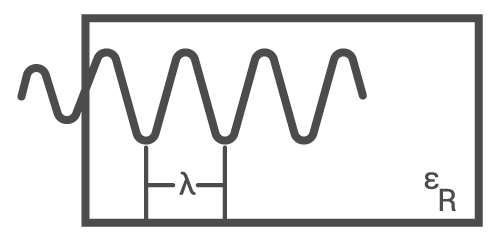# Wavelength (TEM) Calculator

## This calculator determines the wavelength of a signal given the frequency and dielectric constant.

mm

### Overview

A signal's wavelength inside a waveguide is dependent on the medium inside the waveguide. This calculator is designed to calculate the signal wavelength in transverse electromagnetic mode if the dielectric constant or the velocity of propagation is known. Note that the results are presented in millimeters.### Equation

$$\lambda = \frac{c}{f \sqrt{\epsilon_{R}}}$$

or

$$\lambda = v_{p}\frac{c}{f}$$

Where:

$$\lambda$$ = signal wavelength

$$c$$ = speed of light  = 3 x 108 m/s

$$\epsilon_{R}$$ = dielectric constant

$$f$$ = signal frequency

$$v_{p}$$ = velocity of propagation

### Applications

In free space or a vacuum, radio signals travel at the speed of light c (300,000,000 meters per second) because there is nothing that can impede their motion. In other mediums, radio signals tend to travel slower and have a velocity of propagation that is often presented as a percentage over that of the speed of light. The velocity of propagation is found to be related to the dielectric constant of the medium through the equation:

$$v_{p} = \frac{1}{\sqrt{\epsilon_{r}}}$$

The medium inside a waveguide is mostly air and so the speed of the radio signal propagating through it is determined by the dielectric constant of air. The dielectric constant of air at a pressure of 1 atm is 1.00059. This would result in a velocity of propagation equal to 0.99975 (using the formula above). Thus, a radio signal travels 0.9975 slower inside an air-filled waveguide compared when it is travelling in free space.

Textbook - Waveforms•GabNet November 03, 2018